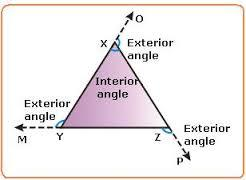Exterior Angles & Angle Sum: Triangle
4 years ago
jenbarker
Save
Edit
Host a game
Live GameLive
Homework
Solo Practice
Practice15 QuestionsShow answers
• Question 1
300 secondsQ. Solve for x.
-11
9
-9
10
• Question 2
300 secondsQ. Solve for x.
8
-10
-7
6
• Question 3
300 secondsQ. Solve for x.
5
12
-7
-6
• Question 4
300 secondsQ. Solve for x.
4
6
7
-10
• Question 5
300 secondsQ. Solve for x.
-11
-12
-6
11
• Question 6
300 secondsQ. Solve for x.
7
9
-11
-6
• Question 7
300 secondsQ. Find the measure of angle A.
20 degrees
22 degrees
21 degrees
100 degrees
• Question 8
300 secondsQ. Find the measure of angle A.
21 degrees
31 degrees
128 degrees
24 degrees
• Question 9
300 secondsQ. Find the measure of angle A.
30 degrees
160 degrees
140 degrees
32 degrees
• Question 10
300 secondsQ. Find the measure of angle A.
132 degrees
111 degrees
28 degrees
22 degrees
• Question 11
300 secondsQ. Find the measure of the indicated angle.
35 degrees
40 degrees
25 degrees
30 degrees
• Question 12
300 secondsQ. Find the measure of the indicated angle.
38 degrees
43 degrees
22 degrees
76 degrees
• Question 13
300 secondsQ. Find the measure of the indicated angle.
87 degrees
82 degrees
83 degrees
72 degrees
• Question 14
300 secondsQ. Find the measure of the indicated angle.
145 degrees
24 degrees
20 degrees
21 degrees
• Question 15
300 secondsQ. Find the measure of the indicated angle.